### Lazuli and the Numbers 7+

Three different degrees of difficulty each
Over 400 tasks
Foundations of counting, characteristics, quantities, symmetry, memory, perception, and geometry
For ages approx. seven years and over

### Peacocks

Instruction e.g.: "Which nest has more eggs?"
Basic Mathematical Skills:
Basic Non-Numerical Skills: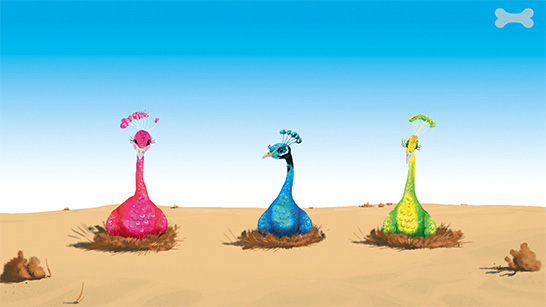### Balancing Act

Instruction e.g.: "Remember the numbers in this order!
Tap on all numbers in this order!"
Basic Mathematical Skills:
Basic Non-Numerical Skills: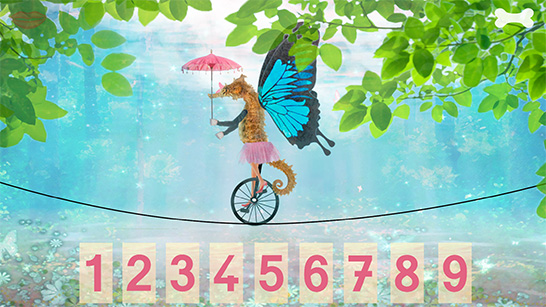### Buttons

Instruction e.g.: "Push the red button in the upper left corner!"
Basic Non-Numerical Skills:### Miró

Instruction e.g.: "These two pictures have one difference. Find the difference and tap on it!"
Basic Mathematical Skills:
Basic Non-Numerical Skills: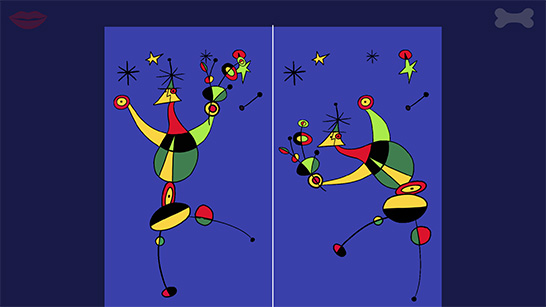### Lollipops

Instruction e.g.: "The lollipops are equally divided up among the children. How many lollipops does each child get?
Tap on the correct number!"
Basic Mathematical Skills: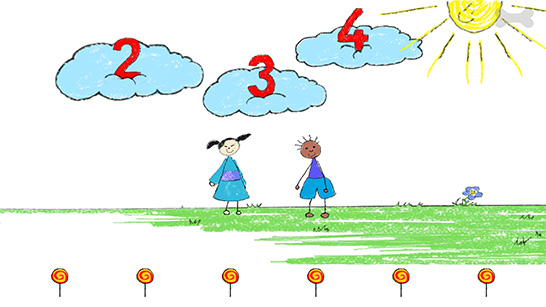### Logical Sequence

Instruction e.g.: "Below there are a few numbers in a certain order. One number is missing from this row. Push this number to the correct place!"
Basic Mathematical Skills:
Basic Non-Numerical Skills: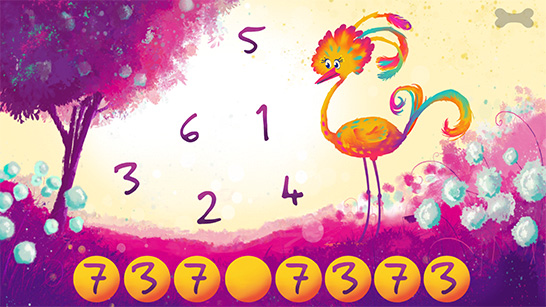### Water

Instruction e.g.: "Tap on the numbers in the correct order from 1 to 5!"
Basic Mathematical Skills: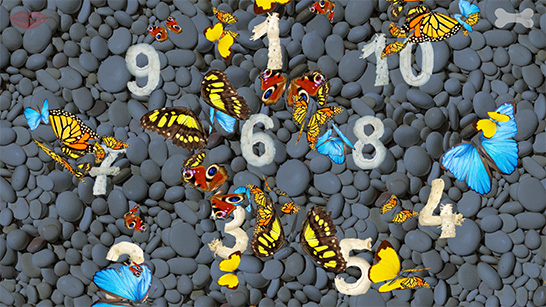### Octopus

Instruction e.g.: "Tap on the largest number!"
Basic Mathematical Skills: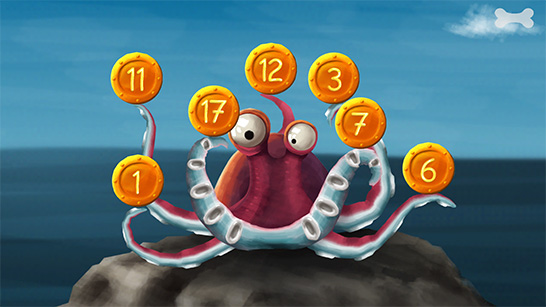### Arithmetic

Instruction e.g.: "What is the answer here?
Tap on the correct number!"
Basic Mathematical Skills: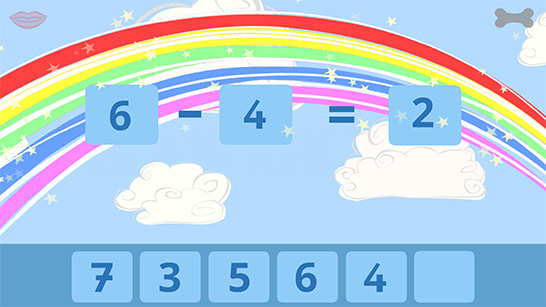### Birds

Instruction e.g.: "How many birds flew away?
Tap on the correct number!"
Basic Mathematical Skills:
Basic Non-Numerical Skills: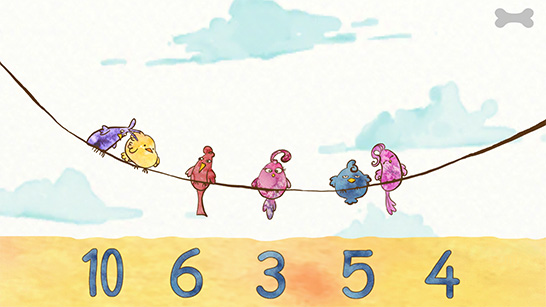### Niki

Instruction e.g.: "Look carefully at this picture! Try to remember it!
Where is the picture now?"
Basic Mathematical Skills:
Basic Non-Numerical Skills: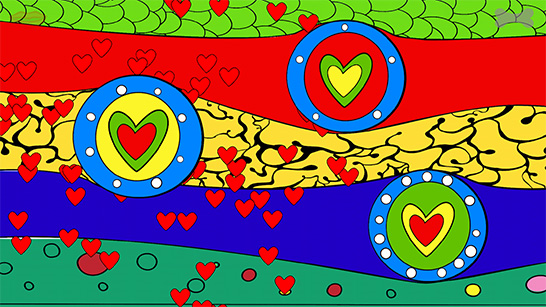### Tree

Instruction e.g.: "Tap on 2 times 3 buds!"
Basic Mathematical Skills:### Rabbit

Instruction e.g.: "Which flowers are in the circle, but not in the triangle?"
Basic Mathematical Skills:
Basic Non-Numerical Skills: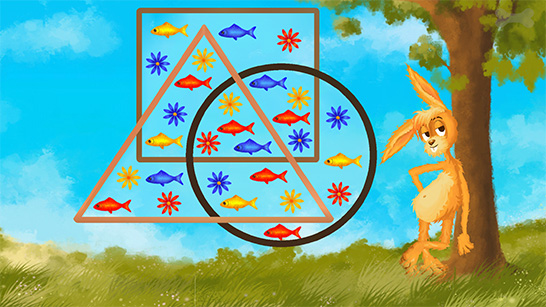### Dots

Instruction e.g.: "If the dots doubled, how many dots would there be?"
Basic Mathematical Skills: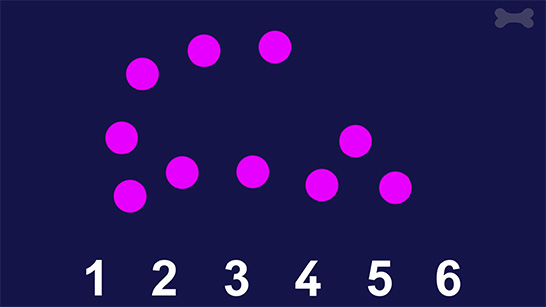### Sheep

Instruction e.g.: "Which group of sheep must go to the meadow below, in order for there to be the same number of black and white sheep on the meadow?
Tap on the top of the correct field!"
Basic Mathematical Skills:
Basic Non-Numerical Skills: